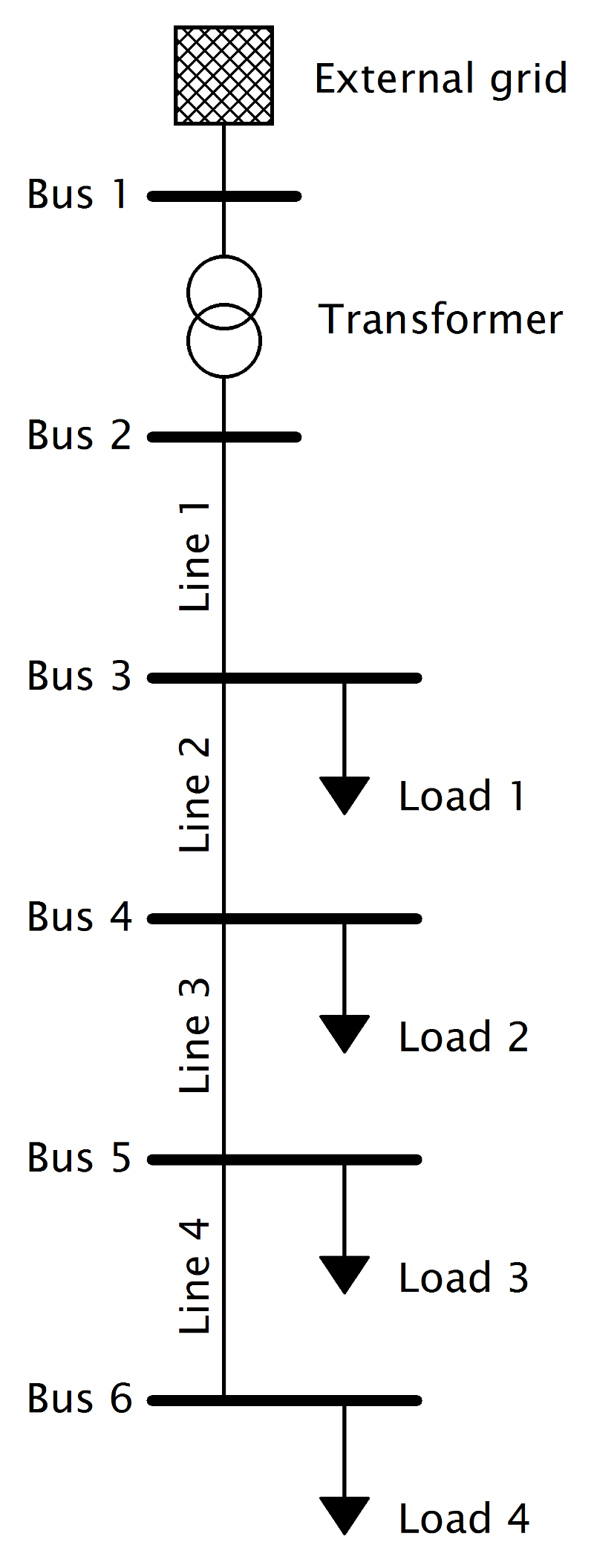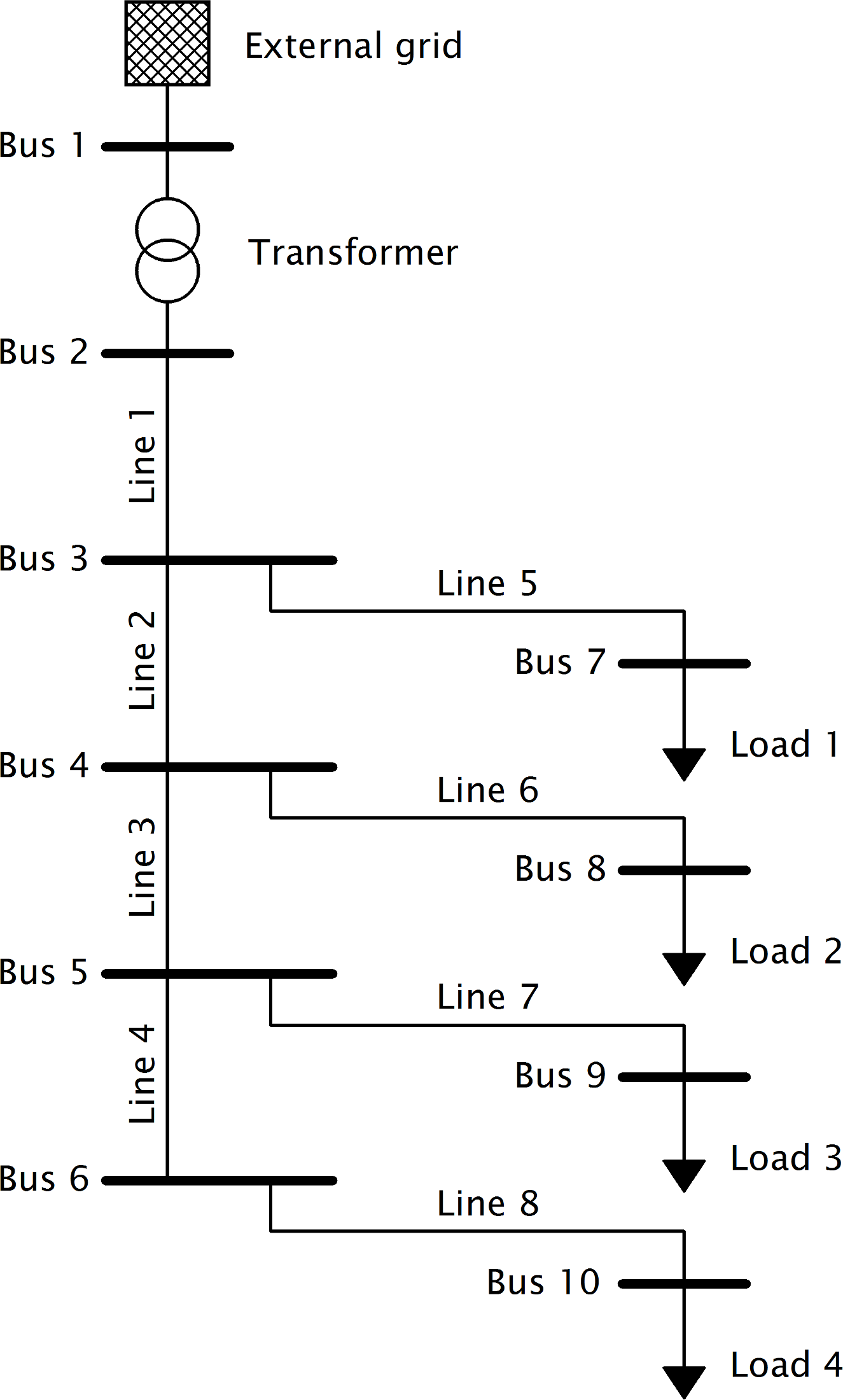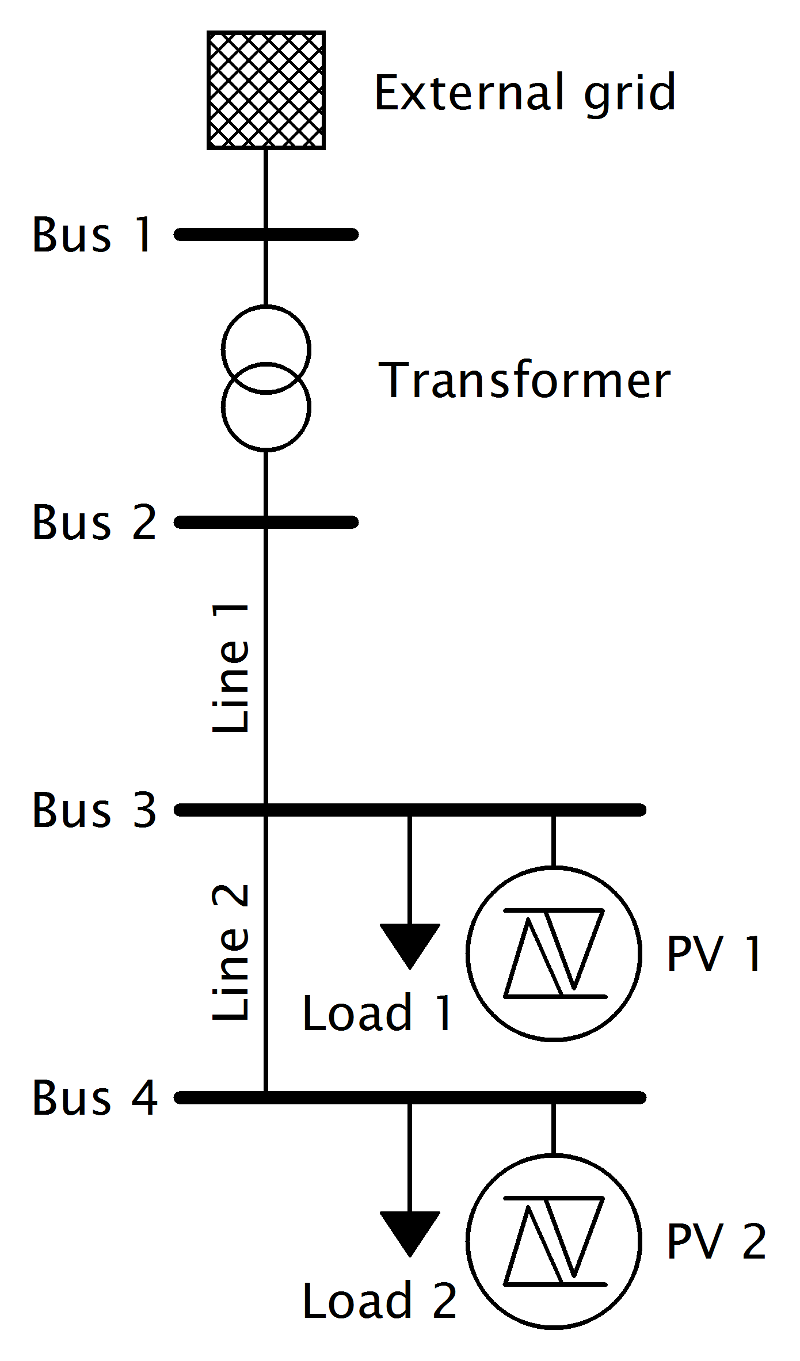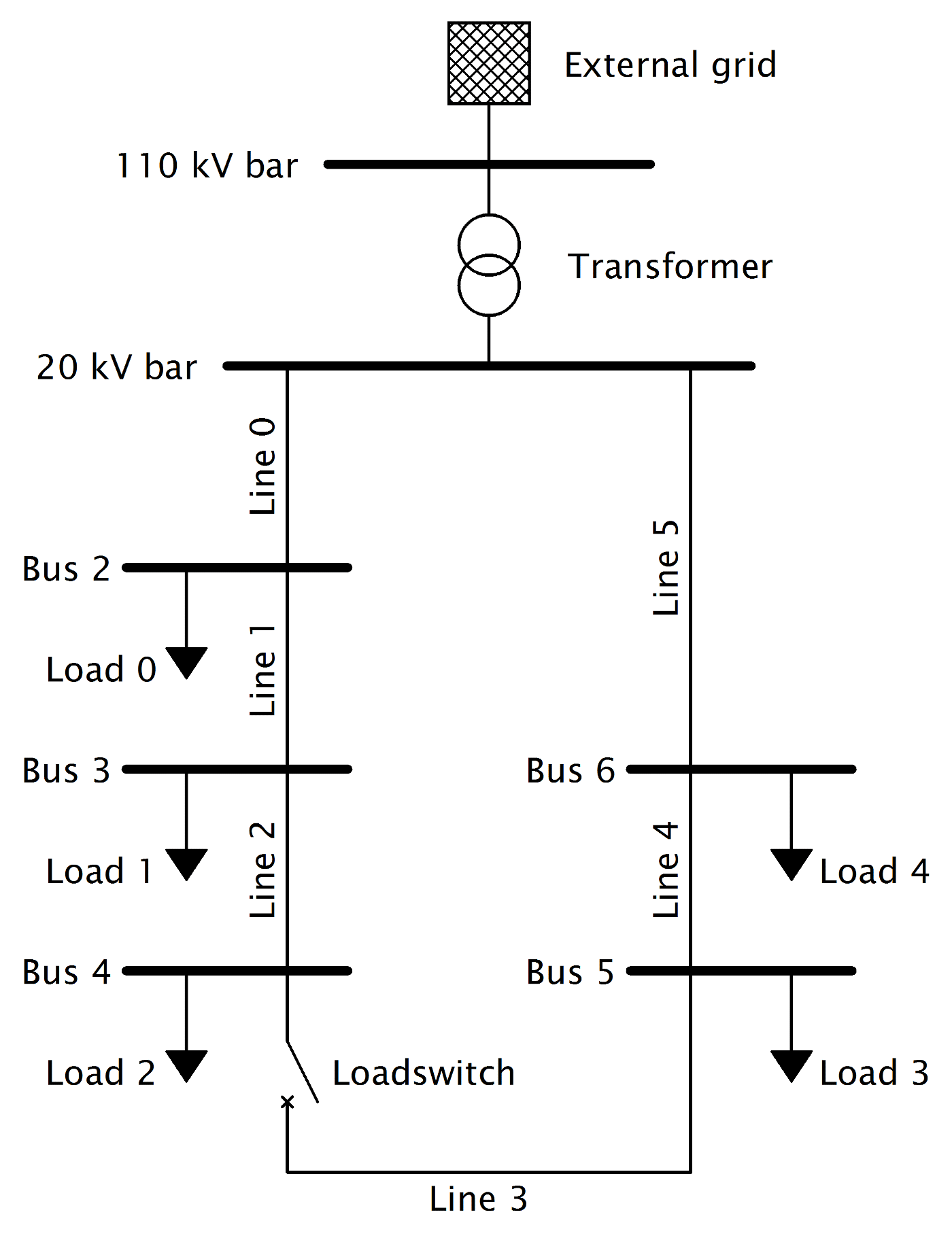# Simple pandapower Test Networks¶

pandapower.networks.panda_four_load_branch()

This function creates a simple six bus system with four radial low voltage nodes connected to a medium voltage slack bus. At every low voltage node the same load is connected.

OUTPUT:

net - Returns the required four load system

EXAMPLE:

import pandapower.networks as pn## Four loads with branches out¶

pandapower.networks.four_loads_with_branches_out()

This function creates a simple ten bus system with four radial low voltage nodes connected to a medium voltage slack bus. At every of the four radial low voltage nodes another low voltage node with a load is connected via cable.

OUTPUT:

net - Returns the required four load system with branches

EXAMPLE:

import pandapower.networks as pn## Four bus system¶

pandapower.networks.simple_four_bus_system()

This function creates a simple four bus system with two radial low voltage nodes connected to a medium voltage slack bus. At both low voltage nodes the a load and a static generator is connected.

OUTPUT:

net - Returns the required four bus system

EXAMPLE:

import pandapower.networks as pn

net_simple_four_bus = pn.simple_four_bus_system()## Medium voltage open ring¶

pandapower.networks.simple_mv_open_ring_net()

This function creates a simple medium voltage open ring network with loads at every medium voltage node. As an example this function is used in the topology and diagnostic docu.

OUTPUT:

net - Returns the required simple medium voltage open ring network

EXAMPLE:

import pandapower.networks as pn

net_simple_open_ring = pn.simple_mv_open_ring_net()